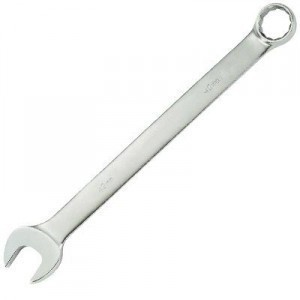# Wrench Dimensions

Wrenches are either in metric or standardSAE sizes. Refer to the proceeding information to make conversion of the measurements easier.

### The S.A.E. Standard Wrench Sizes and Metric Equivalents

The SAE size 5/32" is equal to 4 mm. SAE size 3/16 is equal to 5 mm and size 7/32 to 6 mm. The 1/4 wrench is equal to 7 mm. 5/16 is the same as 8 mm. The 11/32 size is equivalent to 9 mm. The 3/8 wrench is the same as 10 mm.

The 13/32" is equal to 11 mm. The 15/32 is the same as 12. The 1/2 wrench is equal to 13 mm, while the 17/32" is the same as 14 mm. Size 9/16 is equivalent to 14, while 21/32 is equal to 17 mm.

### Other Wrench Sizes and Their Equivalents

Size 11/16 is equivalent to 18 mm; 23/32 is equal to 19 mm; the 25/32 SAE size is about the same as 20 mm. The 13/16 wrench is equal to 21 and the 27/32 wrench size is nearly the same as the 22 mm wrench. The 7/8 wrench is 23 mm and the 15/16 the same as 24.

The conversions given here are not entirely precise owing to unit differences. However they are close enough to give a buyer or user an idea of the sizes. If you aren’t sure, it’s a good idea to ask the person in the hardware store. Depending on your location, other measurement units may be used.

### Wrench Sizes in Inches (the SAE)

5/32 is equal to 0.156 inches; 3/16 is the same as 0.188"; 7/32 is equal to 0.219; the 1/4 wrench is the same as 0.250, while the 9/32 is equal to 0.281 inches.

The 5/16" wrench measures 0.313 inches. The 11/32 is equivalent to 0.344 and the 3/8, 0.375 inches. Other measurements are: 13/32 (0.406"); 7/16 (0.438"), 15/32 (0.469"), and 1/2 (0.500").

The 17/32 is equal to 0.531; 9/16 is equal to 0.563 while the 19/32 wrench is 0.594. 21/32 is 0.656" and 11/16, 0.688". The 23/32 is equal to 0.719; 3/4 is the same as 0.750". 25/32 is equal to 0.781; 13/16 to 0.813 inches, and 27/32 to 0.844. The 7/8 wrench is equal to 0.875.

### Wrench Sizes in Inches (Metric Wrench)

4 mm is equal to 0.157 inches, while 5 is equal to 0.197". Size 6 mm is the same as 0.236; 7 is 0.276" and 8 mm, 0.315". 9 mm is the same as 0.354 inches and 10 mm, 0.394 niches. 11 mm is equivalent to 0.433 and 12 mm to 0.472".

13 mm is equal to 0.512. Other sizes are: 14 mm (0.551"), 15 (0.591), 16 (0.630) and 17 (0.669). 18 mm is equal to 0.709" and 19 mm to 0.748. Size 20 is the same as 0.787; 21 mm is equal to 0.827"; 22 is the same as 0.866; 23 is the same as 0.9055 and 24, 0.945.

Most hardware stores have different wrench sizes available. Just inform the person what you need to do. They can tell you what wrench you’ll need to use.